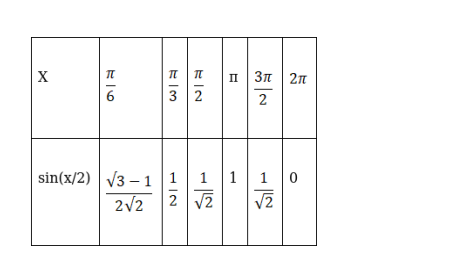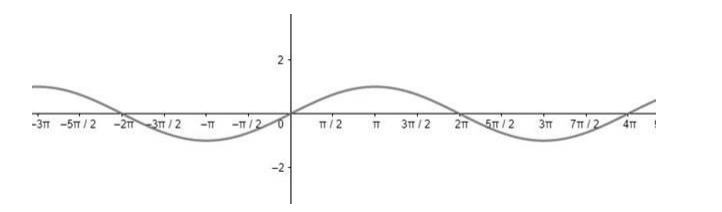# Draw the graph of each of the following functions:`
Question:

Draw the graph of each of the following functions:

$\sin \frac{x}{2}$

Solution:

To draw the graph of the curve sin(x/2) assume some standard angle measures which will help in locating the points and drawing the curve.Therefore, the graph of curve 2cos(3x) can be drawn asHere, the frequency of the function sin(x) is decreased by 0.5 times.